This document contains details omitted from the main paper.

# 1. Discussion of the Tarallo Water Diversion Fake

## 1.1. Summary

Proposed by : Flavio Tarallo (as reported by Rothwell) : Vortex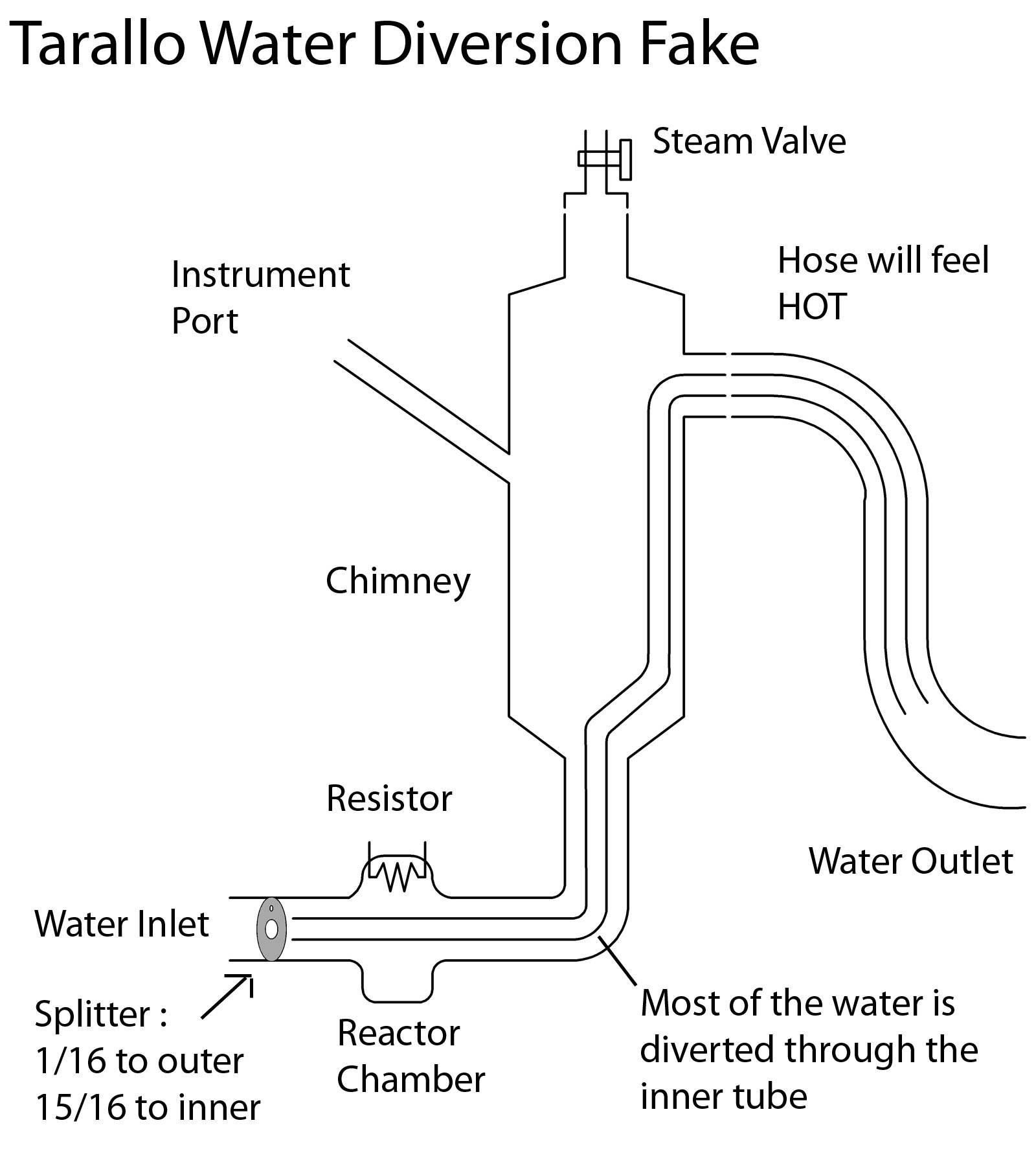This is a very serious fake. It would possibly not have been detected by the January experiment or the February experiment.

ALL of the measurements in ALL of the experiments were done via the instrument port in the chimney, and would only have measured the temperature and steam dryness in the outer compartment.

The water sent through the "reactor chamber" is simply heated by a resistor, powered from the control box.

The observed "temperature profiles" could easily be accomplished by changing the power sent to the resistor: the connection between the control box and the main unit was NOT measured.

## 1.2. Vortex Discussion about the Torelli Fake

Rothwell Okay, what insulator would you use inside the pipe? You need something thin enough to allow the water through and yet effective at 1 L/s with a 31°C temperature difference for the apparent 130 kW heat in the Feb. 10 test.

The duration of the 130kW was unspecified, so I'll stick with the March experiment. If we use the above diagram, with a 3 cm diameter outer section, and put a 1cm diameter bypass through the device, with a diversion ratio of 1/16 to 15/16 (roughly equal to the given power ratio). The bypass tube could be rubber (a reasonable insulator).

Thermal Conductivity : watts / m.K Rubber is 0.16 :

```Area  A =  2 *  3.1415 * 0.500 * 100.000  = 314.15000000 cm2
Thickness Y : 0.500 cm
dT = 100 - 20 = 80.000
k of rubber = 0.160
Watts = k * dT * A / Y
= 0.160 * 80.000  * 0.03141500 / 0.005
=  80.4224
```
Even this value could be handled in the March fake, which had 300W of electrical power available. : the amount of water diverted would have to take this loss into account. Since the water would warm up as it passes through the reactor the average dT would actually be less than that just calculated.

The bypass could be engineered to minimise this loss. It could be arranged to be in contact with the outer wall, so the effective transfer area would be reduced. Wrap it in a (waterproofed) Silica Aerogel (0.004 to 0.04) and the problem goes away.

Since the horizontal arm was not unwrapped the bypass tube could have been completely OUTSIDE the reactor chamber section, entering at the bottom of the chimney. Only about 20 cm would be inside the device, so the power loss would be less than 20 W.

Why wouldn't Levi et al. notice that they cannot insert thermocouples more than a faction of the way into the hose? (If they could insert it and it did not penetrate the barrier, it would block the middle channel.)

They didn't insert it into the hose. They inserted it into the instrument port.

The above diagram shows the bypass tube in the center of the chimney. It could be at the side (or even hidden by a false wall near the instrument port).

Stephen A. Lawrence : Vortex

It's totally ruled out if the effluent is observed to be steam and the output temperature is claimed to be roughly 100C. Whether wet steam or dry steam, if it's coming out as steam, then the outlet temperature is at least 100C, and the placement of the temperature probe is irrelevant. During the first test, back in ... uh ... January?, the effluent was observed to be steam during at least part of the run, and this effect couldn't have been an issue.

The probe was always placed in the outer "Hot" compartment. Nobody reported in January that they checked for steam coming OUT of the hose. Nobody reported in February that they measured the temperature of the water coming out of the hose.

Rothwell However, in this test, the outlet temperature is measured in the "chimney." That is large enough to implement this trick fairly easily, with steam passing the thermocouple, well insulated from the stream of cold water. However, the outlet tube would be close to tap water temperature, which would be a dead giveaway. I am assuming someone had the sense to hold a hand near it or touch it for a moment. I would do that the moment I saw the test.

To make the black tube hot, you have to imagine there is barrier within the hose that allows a thin layer of steam to pass on the outside at a high temperature without being cooled by the water in the center. It is moving at 1.7 ml/s. From the photo I suppose that hose is 1 cm OD and 0.8 cm ID, which is to say a volume of 0.5 cm^3 per centimeter of hose. So if the water is liquid, it is moving about 4 cm per second. It would cool down a short distance from the chimney. Real steam moving out of that hose would reach a lot farther than 4 cm per second, and heat the entire hose. There would still be a lot of steam coming out of the end of the hose in the bathroom.

The output tube used in March is considerably thicker than 1 cm. Looks about 2 cm at least. The fake I'm proposing does have a coaxial tube for some arbitrary distance in the output hose, so the outside of the hose will indeed feel hot. The thermal conductivity analysis above could be applied here.

Thinking aloud here ... first see how long it takes for the diverted water to go through the tube.

```
flow 6.470 L/Hr = 1.797 cc/sec  Hot: 0.112 cc/sec Cold: 1.685 cc/sec
inner tube radius : 0.500 cm  length : 100.000  cm  volume : 78.538 cc
time in reactor : 46.613 secs
```

Y

# 2. March 2011 Experiment : Measurements

A new test had just been released, with pictures of a smaller 5kW device with and without shielding and insulation.

## 2.1. Photos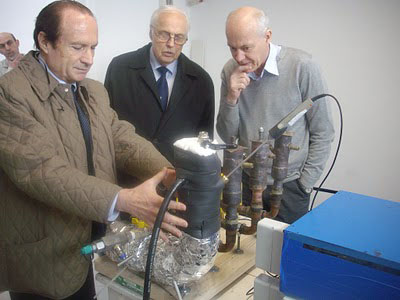Fig 5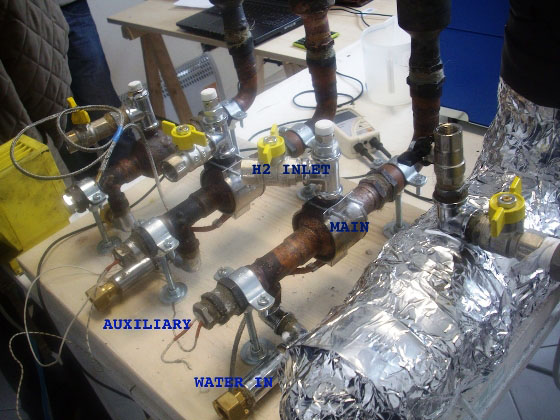Fig 2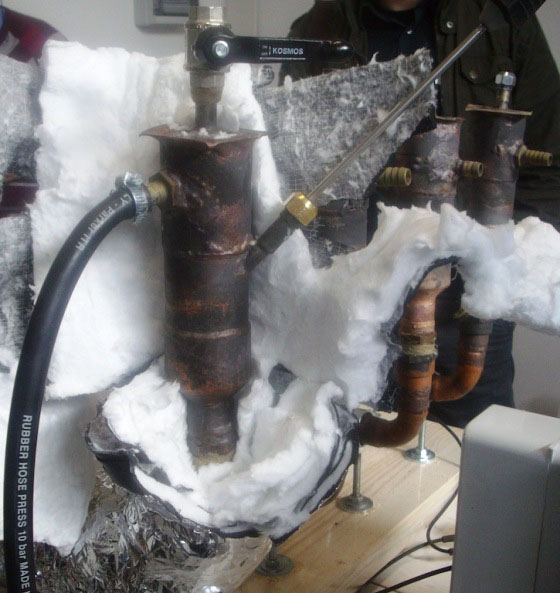Fig 4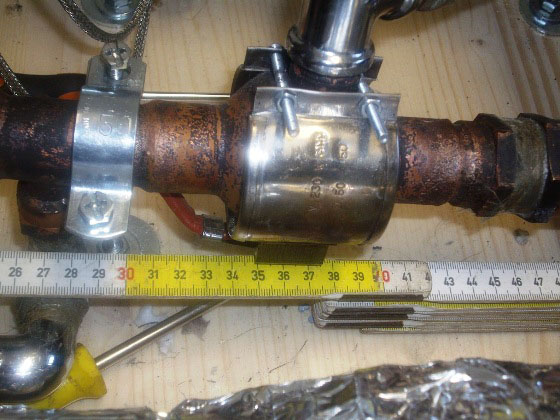Fig 3

Measurement of Sizes from the Photos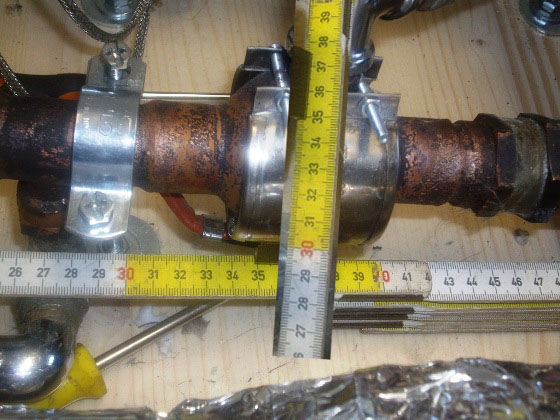Fig E
Ruler rotated in photoshop

The total volume of the REACTOR was estimated as a sphere::

Reactor Sphere diameter: 7.00 cm
volume: 179.59 cm3 = 0.1796 L

Since the horizontal arm was NOT inspected, I have estimated its volume: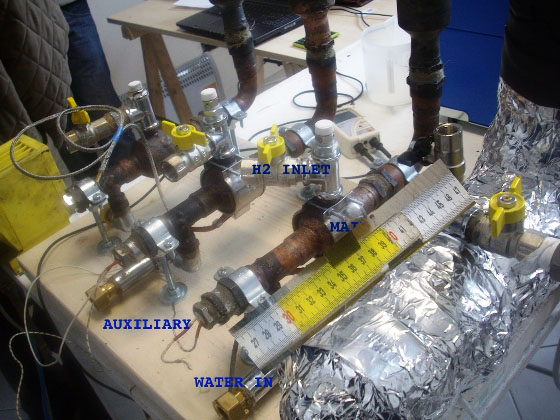Fig F1

Scale ruler from fig 3 onto Fig 2 = 10cm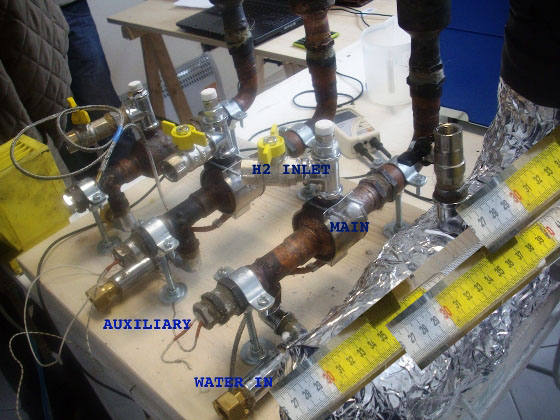Fig F2

Repeat for Length of Horizontal arm = 30cm (Any foreshortening would over-estimate the length.)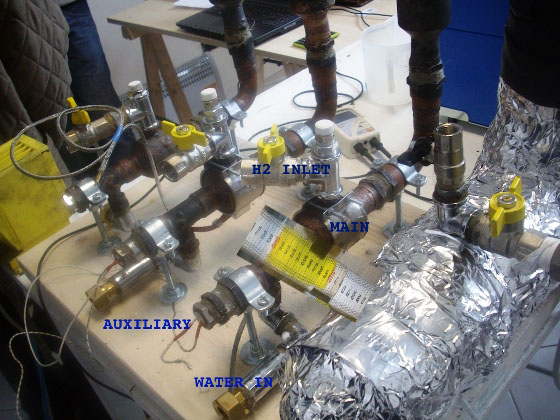Fig F3

Scale rule to diameter of reactor = 7 cm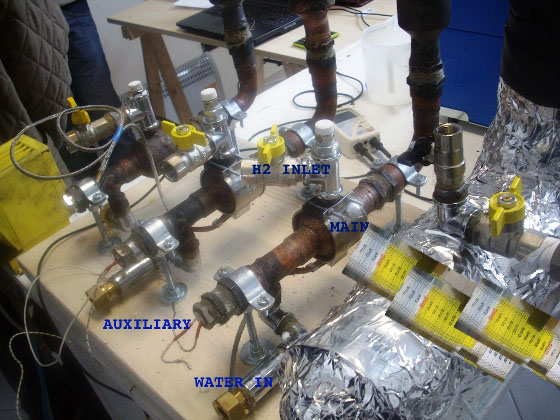Fig F4

Step and Repeat for diameter of horizontal arm = 25cm

Horizontal Arm : cylinder, length: 30.00 cm diameter: 25.00 cm
volume: 14725.78 cm3 = 14.7258 L

# 3. Calculations

## 3.1. Wiki Energy Densities

The Wiki of Energy Densities doesn't have entries for all cases where (for instance) Hydrogen is used with Compressed or Liquid Oxygen.

This section calculates how much the "wiki" Energy Densities by Mass and by Volume are reduced if the available space has to be shared between the Hydrogen and Oxygen.

## 3.3. Atoms and Molecules

```	H    mass 1.0071
H2   mass 2.0142
Gas          Density : 8.988E-5 kg/L
Compressed   Density : 0.046647636700649 kg/L  (700 Bar)
Liquid       Density : 0.07099 kg/L

O    mass 15.999
O2   mass 31.998
Gas          Density : 0.001429 kg/L
Compressed   Density : 0.74164967562558 kg/L  (700 Bar)
Liquid       Density : 1.141 kg/L

B    mass 10.811
Solid  Density : 2.52 kg/L```

## 3.4. Compressed hydrogen + Compressed Oxygen

Title: AB[CH-CO] Compressed Hydrogen/Compressed Oxygen
```Formula:  2 H2  + O2  ===> 2  H2O
H2 (Compressed H2)
O2 (Compressed O2)
H2 mass :   2 *  2.014 =  4.028
O2 mass :   1 * 31.998 = 31.998
Total mass :   36.026
H2 mass fac :  4.028 /  36.026 =  0.112
O2 mass fac : 31.998 /  36.026 =  0.888
Volume : mass / density
H2 volume :   4.028 /  0.047 =  86.358
O2 volume :  31.998 /  0.742 =  43.144
Total vol :  129.502
H2 vol fac : 86.358/129.502 =  0.667
O2 vol fac : 43.144/129.502 =  0.333
```

## 3.5. Liquid hydrogen + Liquid Oxygen

Title: AB[LH-LO] Liquid Hydrogen/Liquid Oxygen
```Formula:  2 H2  + O2  ===> 2  H2O
H2 (Liquid H2)
O2 (Liquid O2)
H2 mass :   2 *  2.014 =  4.028
O2 mass :   1 * 31.998 = 31.998
Total mass :   36.026
H2 mass fac :  4.028 /  36.026 =  0.112
O2 mass fac : 31.998 /  36.026 =  0.888
Volume : mass / density
H2 volume :   4.028 /  0.071 =  56.746
O2 volume :  31.998 /  1.141 =  28.044
Total vol :  84.790
H2 vol fac : 56.746/84.790 =  0.669
O2 vol fac : 28.044/84.790 =  0.331
```

## 3.6. Boron + Compressed Oxygen

Title: AB[B-CO] Boron/Compressed Oxygen
```Formula:  4 B  +  3 O2  ===> 2  B2O3
B (Solid B)
O2 (Compressed O2)
B mass :   4 * 10.811 = 43.244
O2 mass :   3 * 31.998 = 95.994
Total mass :   139.238
B mass fac : 43.244 /  139.238 =  0.311
O2 mass fac : 95.994 /  139.238 =  0.689
Volume : mass / density
B volume :  43.244 /  2.520 =  17.160
O2 volume :  95.994 /  0.742 =  129.433
Total vol :  146.593
B vol fac : 17.160/146.593 =  0.117
O2 vol fac : 129.433/146.593 =  0.883
```

## 3.7. Boron + Liquid Oxygen

Title: AB[B-LO] Boron/Liquid Oxygen
```Formula:  4 B  +  3 O2  ===> 2  B2O3
B (Solid B)
O2 (Liquid O2)
B mass :   4 * 10.811 = 43.244
O2 mass :   3 * 31.998 = 95.994
Total mass :   139.238
B mass fac : 43.244 /  139.238 =  0.311
O2 mass fac : 95.994 /  139.238 =  0.689
Volume : mass / density
B volume :  43.244 /  2.520 =  17.160
O2 volume :  95.994 /  1.141 =  84.131
Total vol :  101.292
B vol fac : 17.160/101.292 =  0.169
O2 vol fac : 84.131/101.292 =  0.831
```

## 3.8. Magnesium and Steam

Reactions of Metals and Water

Magnesium combines with STEAM to produce Magnesium Oxide and Hydrogen.

```Mg + H2O ==>MgO + H2 dH  -360 kJ/mol  The hydrogen can then be burned with Air or Oxygen to produce water.
H2O: H2 (g) + 1/2 O2 (g) ==> H2O (l); dH = -285.8 kJ/mol
(Remove Latent Heat 41 kJ/mol = -285.8 + 41 kJ/mol )Check math by comparing liquid H /External O2Total (from H2) is -286 kJ/mol.Atomic weight: 2.01 g/mol.Density (L): 0.0710 g/cm3Energy by mass : ( 286 kJ/mol)/(2.01g/mol )     =  142 kJ/g  =  142 MJ/kgEnergy by volume: 10.1 MJ/LComparison to Wiki values

Energy by Mass
Energy by Volume

Wiki
143.000
10.100

Calculation
141.893
10.100

Total (from Mg/Steam + H/O) is -604.8 kJ/mol.
Atomic weight    : 24.305 g/mol
Density          : 1.738 g/cm3
Energy by mass   :  ( 605 kJ/mol) / (24.305 g/mol)  = 24.9 kJ/g
= 24.9 MJ/kg
Energy by volume : 43.2 MJ/L
```
```
Total (from Ni/H) is -4.85 kJ/mol.
Atomic weight    : 58.6934 g/mol
Density          : 8.908 g/cm3
Energy by mass   :  (4.85 kJ/mol) / (58.6934 g/mol)  = 0.0826 kJ/g
= 0.0826 MJ/kg
Energy by volume : 0.736 MJ/L
```

## 3.9. Magnesium (+ Steam) + Compressed Oxygen

Title: AB[MG-H2O-O2] Mg/Steam/O2
```Formula:  Mg  +  H2O  ===> H2 THEN H2 + 1/2 O2 ===> H2O
Mg (Solid Mg)
O2 (1/2 O2)
Mg mass :   1 * 24.305 = 24.305
O2 mass :   0.5 * 31.998 = 15.999
Total mass :   40.304
Mg mass fac : 24.305 /  40.304 =  0.603
O2 mass fac : 15.999 /  40.304 =  0.397
Volume : mass / density
Mg volume :  24.305 /  1.738 =  13.984
O2 volume :  15.999 /  1.141 =  14.022
Total vol :  28.006
Mg vol fac : 13.984/28.006 =  0.499
O2 vol fac : 14.022/28.006 =  0.501
```

## 3.10. Embedded Calculations

All calculations in this document are performed with the PHP programming language which generates the document.

Partial PHP Source Code